# The Parabiaugmented Truncated Dodecahedron

The parabiaugmented truncated dodecahedron is the 69th Johnson solid, or J69 for short. It has 52 faces (10 decagons, 2 pentagon, 10 squares, and 30 triangles), 120 edges, and 70 vertices. It is obtained by augmenting two opposite decagonal faces of the truncated dodecahedron with pentagonal cupolae (J5).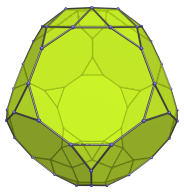If one of the augments is removed, the result is the augmented truncated dodecahedron (J68).

## Projections

Here are some views of the parabiaugmented truncated dodecahedron from various angles:

Projection Description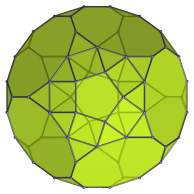Top view.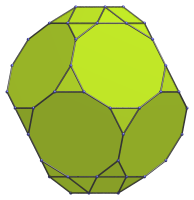Front view.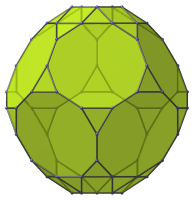Diagonal side view.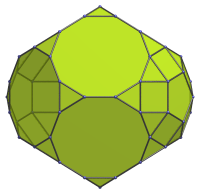Oblique view centered on decagon-decagon edge.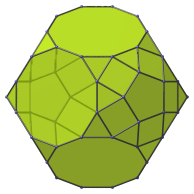Oblique view centered on decagon-triangle edge.

## Coordinates

The Cartesian coordinates of the parabiaugmented truncated dodecahedron with edge length 2 are even permutations of coordinate and all changes of sign of:

• (1, 0, 1+3φ)
• 2, 1, 2φ2)
• (2φ, φ2, φ3)

along with:

• ±((13φ+1)/5, ±1, (6φ+12)/5)
• ±((13φ+6)/5, ±φ, (φ+12)/5)
• ±((18φ+1)/5, 0, (φ+7)/5)

where φ=(1+√5)/2 is the Golden Ratio.

Last updated 18 Jun 2019.